Next: Stationary Hydraulic Jumps Up: Incompressible Inviscid Flow Previous: Sub-Critical and Super-Critical Flow

# Flow over Shallow Bump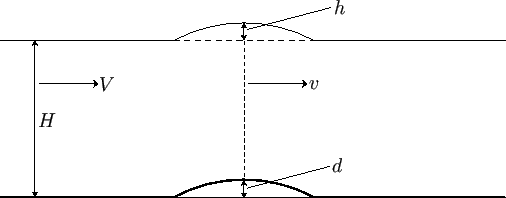Consider a shallow stream of depth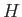, uniform width, and uniform flow velocity. Suppose that there is a very shallow bump of height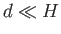on the (horizontal) bed of the stream, as shown in Figure 4.5. Suppose, further, that, at the point where the stream passes over the top of the bump, its velocity is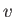, and its surface rises a height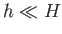above the unperturbed surface.

Fluid continuity yields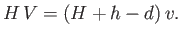(4.29)

Furthermore, application of Bernoulli's equation to a streamline lying on the surface of the water (where the pressure is atmospheric) gives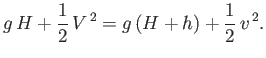(4.30)

The previous equation reduces to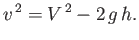(4.31)

Eliminatingbetween Equations (4.29) and (4.31), we obtain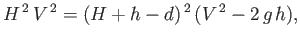(4.32)

which can be rearranged to give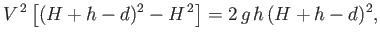(4.33)

or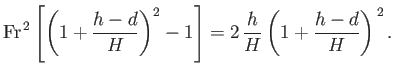(4.34)

Here,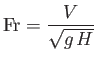(4.35)

is the Froude number of the unperturbed flow. Finally, given that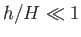and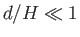, Equation (4.34) reduces to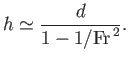(4.36)

It follows, from the previous expression, that if the flow is super-critical, so that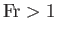, thenis positive. On the other hand, if the flow is sub-critical, so that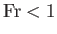, thenis negative. Thus, if a super-critical shallow stream passes over a very shallow bump on its bed then the surface of the stream becomes slightly elevated. On the other hand, if a sub-critical stream passes over such a bump then the surface of the stream becomes slightly depressed. A similar effect occurs when there is a narrowing of the channel in the horizontal direction. A more sophisticated version of the previous calculation, which does not necessarily assume that the stream is shallow, can be found in Section 11.10.Next: Stationary Hydraulic Jumps Up: Incompressible Inviscid Flow Previous: Sub-Critical and Super-Critical Flow
Richard Fitzpatrick 2016-03-31